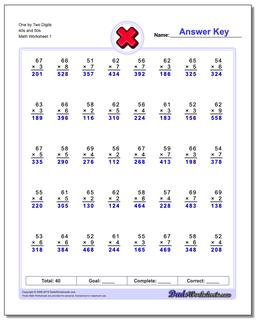# Math Worksheets: Multiplication: Multiplication: One by Two Digits 40s and 50s## One by Two Digits 40s and 50s

PropertyValue
DescriptionOne by Two Digits 40s and 50s: These multiplication worksheets introduce two digit and three digit multiplication problems. Try out this multiplication calculator to see interactively how to do long multiplication.
Resource TypeWorksheet